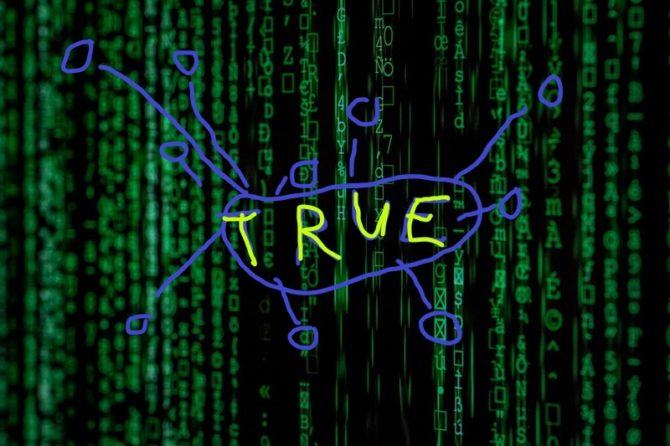# How to use NumPy Boolean Indexing to Uncover Instagram Influencers

This article will give you a practical one-liner solution and teach you how to write concise NumPy code using boolean indexing and broadcasting in NumPy.

## The Basics

NumPy plays an important role in the Python programming language. Not only does it add basic linear algebra functionality to Python, but, with its array data structure, it also provides a better and more convenient way of representing your data sets. In a way, NumPy arrays enrich the basic list data type with additional functionality such as multi-dimensional slicing and convenient indexing.

Have a look at the following code snippet.

```import numpy as np

a = np.array([[1, 2, 3],
[4, 5, 6],
[7, 8, 9]])

indices = np.array([[False, False, True],
[False, False, False],
[True, True, False]])

print(a[indices])
# [3 7 8]
```

We create two arrays “a” and “indices”. The first array contains two-dimensional numerical data – you can think of it as the data array. The second array has the same shape and contains Boolean values – think of it as the indexing array. A great feature of NumPy is that you can use the Boolean array for fine-grained data array access. In plain English, we create a new NumPy array from the data array containing only those elements for which the indexing array contains “True” Boolean values at the respective array positions. Thus, the resulting array contains the three values 3, 7, and 8.

In the following one-liner, you are going to use this feature for miniature social network analysis.

## The Code

We are examining the following problem: “Find the names of the Instagram superstars with more than 100 million followers!”

```## Dependencies
import numpy as np

## Data: popular Instagram accounts (millions followers)
inst = np.array([[232, "@instagram"],
[133, "@selenagomez"],
[59,  "@victoriassecret"],
[120, "@cristiano"],
[111, "@beyonce"],
[76,  "@nike"]])

## One-liner
superstars = inst[inst[:,0].astype(float) > 100, 1]

## Results
print(superstars)
```

You can compute the result of this one-liner in your head, can’t you?

## The Result

The data consists of a two-dimensional array where each row represents an Instagram influencer. The first column states their number of followers (in million), and the second column states their Instagram name. The question is to find the names of the Instagram influencers with more than 100 million followers.

The following one-liner is one way of solving this problem. Note that there are many more alternatives – this is just the one which I found has the least number of characters.

```## One-liner
superstars = inst[inst[:,0].astype(float) > 100, 1]
```

Let’s deconstruct this one-liner in a step by step manner.

First, we calculate a Boolean value whether each influencer has more than 100 million followers:

```print(inst[:,0].astype(float) > 100)
# [ True  True False  True  True False]
```

The first column of the data array contains the number of followers, so we use slicing to access this data (inst[:,0] returns all rows but only the first column). However, the data array contains mixed data types (integers and strings). Therefore, NumPy automatically assigns a non-numerical data type to the array.

But as we want to perform numerical comparisons on the first column of the data array (checking whether each value is larger than 100), we first need to convert the array into a numerical type (for example float).

At this point, we check whether a NumPy array of type float is larger than an integer value. What exactly happens here? You have already learned about broadcasting: NumPy automatically brings the two operands into the same shape. Then, it compares the two equally-shaped arrays element-wise. The result is an array of Boolean values. Four influencers have more than 100 million followers.

We now take this Boolean array as an indexing array to select the influencers with more than 100 million followers (the rows).

`inst[inst[:,0].astype(float) > 100, 1]`

As we are only interested in the names of these influencers, we select the second row as the final result stored in the superstars variable.

The influencers with more than 100 million Instagram followers are:

`# ['@instagram' '@selenagomez' '@cristiano' '@beyonce']`

## What’s next?

Learning NumPy will not only make you a better Python coder, it will also improve your chances to find profitable positions as a data scientist and solve important real-world problems.

To help you increase your value to the marketplace, I’ve written a new NumPy book — 100% based on the proven principle of puzzle-based learning.